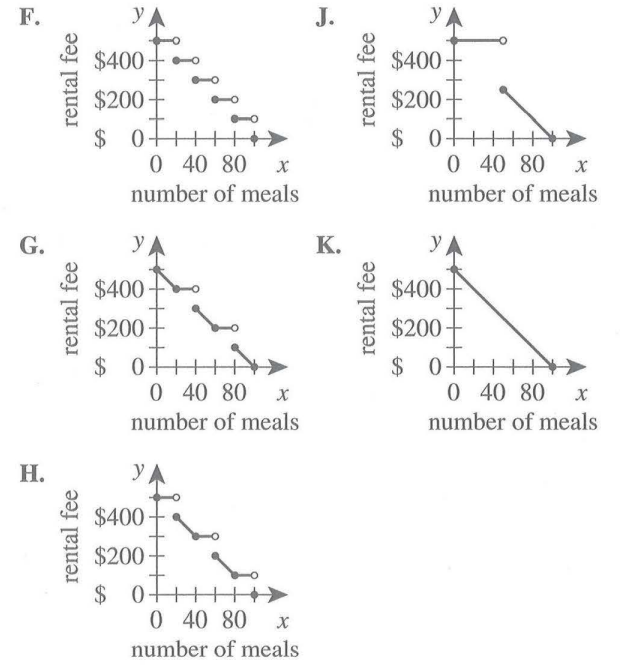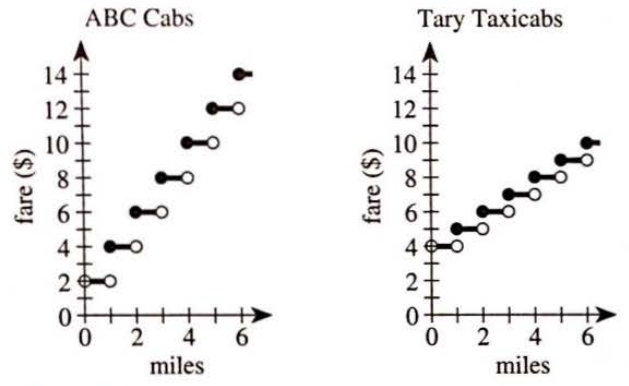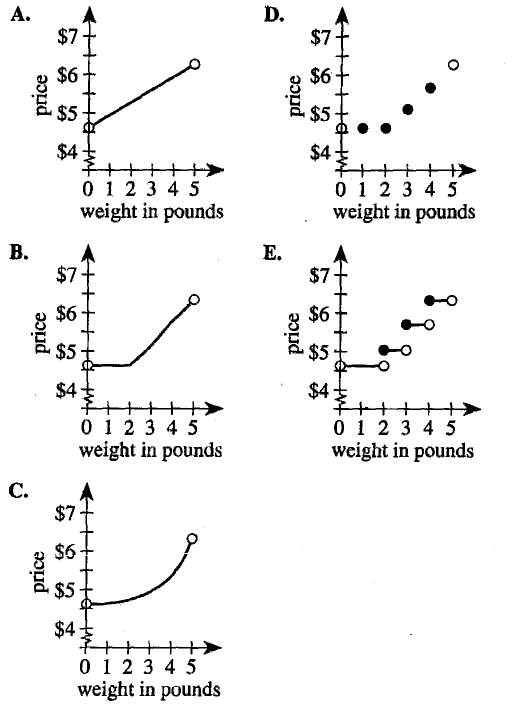If there is one prayer that you should pray/sing every day and every hour, it is the LORD's prayer (Our FATHER in Heaven prayer)
It is the most powerful prayer. A pure heart, a clean mind, and a clear conscience is necessary for it.
- Samuel Dominic Chukwuemeka

For in GOD we live, and move, and have our being. - Acts 17:28

The Joy of a Teacher is the Success of his Students. - Samuel Dominic Chukwuemeka

# Solved Examples on the Graphs of Piecewise FunctionsFor ACT Students
The ACT is a timed exam...60 questions for 60 minutes
This implies that you have to solve each question in one minute.
Some questions will typically take less than a minute a solve.
Some questions will typically take more than a minute to solve.
The goal is to maximize your time. You use the time saved on the questions you solve in less than a minute, to solve the questions that will take more than a minute.
So, you should try to solve each question correctly and timely.
So, it is not just solving a question correctly, but solving it correctly on time.
Please ensure you attempt all ACT questions
There is no negative penalty for a wrong answer.

Solve all questions
Show all work

(1.) ACT The rental fee for the use of a reception hall is $500 To encoorage use of the hall's catering service, the hall's rental fee is reduced by$100 for each group of 20 meals ordered through the hall's catering service.
All 20 meals in a group must be ordered for each reduction in the rental fee.
One of the following graphs shows the hall's rental fee when 0 to 100 meals are ordered.
Which graph is it?Let us represent the information using a Table of Values ... for 0 to 100 meals
Two variables: rental cost and number of meals
The rental cost depends on the number of meals
This implies that:
the rental cost is the dependent variable = y variable
the number of meals is the independent variable = x variable

The Table of Values is:
Number of meals Rental cost ($\$$)$xy0500204004030060200801001000$Based on the graphs, Options: F, G, and H satify the Table of Values However, based on the wording in the question: All 20 meals in a group must be ordered for each reduction in the rental fee: the correct answer is Option F because the increments must be in 20's only (no in-between values) (2.) ACT ABC Cabs and Tary Taxicabs both have an initial fare of a while number of dollars for 1 passenger. The fare increases a whole number of dollars at each whole number of miles traveled. The graphs below show the 1-passenger fares, in dollars, for both cab companies for trips up to 6 miles. When the fares of the 2 cab companies are compared, what is the cheaper fare for a 5-mile trip?$ F.\;\; \$8 \\[3ex] G.\;\; \$ 9 \\[3ex] H.\;\; \$10 \\[3ex] J.\;\; \$ 11 \\[3ex] K.\;\; \$12 \\[3ex]$

The value in the y-axis (the fare) for the closed circle value in the x-axis (the miles)

$For\;\;a\;\;5-mile\;\;trip: \\[3ex] ABC\;\;Cabs:\;\;fare = \$12 \\[3ex] Tary\;\;Taxicabs:\;\;\; fare = \$9 \\[3ex] Cheaper\;\;fare = \$9 $(3.) ACT The table below gives the price to mail a single package through the United States Postal Service on August 30, 2007. The price depended on the weight of the package.  Weight in pounds Price 0 < x < 2 2 ≤ x < 3 3 ≤ x < 4 4 ≤ x < 5$4.60 $5.05$5.70 $6.30 Which of the following graphs best represents this information?This is a Piecewise Function where the values of the output for each domain are contants It is very evident that Option E is the correct option because it is a Piecewise Function graph that denotes the fact that each output is a constant. But if you do want me to explain further: Remember that the closed circle value is the value that actually corresponds to each output So,$x = 2$corresponds to$y = \$5.05$
Looking at the graphs in Options A, B, C, and D; when $x = 2$, $y \lt 5.05$
So, those options are eliminated.
When you review the graph in Option E; that graph corresponds to the Table of Values
When $x = 2$, $y = 5.05$
When $x = 3$, $y = 5.70$
When $x = 4$, $y = 6.30$
(4.) ACT Which of the following is the graph of the function f(x) defined below?

$f(x) = \begin{cases} x^2 - 2 \enspace \text{for} \enspace x \le 1 \\[3ex] x - 7 \enspace \text{for} \enspace 1 \lt x \lt 5 \\[3ex] 4 - x \enspace \text{for} \enspace x \ge 5 \end{cases} \\[7ex]$Let us draw the Table of Values for each function/piece
Then, we shall answer the question by going through each option and eliminating the incorrect options until we find the correct one

 $x$ $-2$ $-1$ $0$ $1$ $y$ $2$ $-1$ $-2$ $-1$ defined

 $x$ $1$ $2$ $3$ $4$ $5$ $y$ $-6$ $-5$ $-4$ $-3$ $-2$ undefined undefined

 $x$ $5$ $6$ $7$ $8$ $y$ $-1$ $1$ $2$ $3$ defined

Option F.
First Piece: when $x = 1$, $y = -3$
This is incorrect...as seen from the Table.

Option J.
First Piece: when $x = 1$, $y = 0$
This is incorrect...as seen from the Table.

Option G.
First Piece: when $x = -2$, $y = 0$
This is incorrect...as seen from the Table.

Option K.
This is the correct option because all the Tables of Values corresponds to the graph
This includes where the values are defined (closed circles) and undefined (open circles)
You may STOP here (or if you have time, check the last option)

Option H.
First Piece: when $x = 0$, $y = 0$
This is incorrect...as seen from the Table.
(5.)

(6.)

(7.)

(8.)

(9.)

(10.)